#### Analysis of pericyclic reactions, Chemistry tutorial

Introduction

The Diels-Alder reaction is one kind of a broader class of reactions, which are recognized as pericyclic reactions. In the year 1965 two other Nobel laureates, Robert B.Woodward and Roald Hoffmann, published a series of short communications in that they presented a theoretical basis for these well known, but poorly understood pericyclic reactions. Their theory is said orbital symmetry theory. Consequently other chemists published alternative interpretations of pericyclic reactions, one called frontier orbital theory, and another named aromatic transition state theory. All of these theories are based upon MO theory. In this chapter we will utilize the Diels-Alder reaction to illustrate aspects of each of these theories.

Analysis of Pericyclic reactions

Principal techniques of analyzing pericyclic reactions are the orbital correlation diagram technique, the frontier molecular orbital (FMO) approach, and the Mobius-Huckel transition state aromaticity approach.

Orbital correlation diagram method

The original approach of Woodward and Hoffmann involved construction of an "orbital correlation diagram" for each type of pericyclic reaction. The symmetries of the appropriate reactant and product orbitals were matched to determine whether the transformation could precede without symmetry imposed conversion of bonding reactant orbitals to anti bonding product orbitals. If the correlation diagram indicated that the reaction could occur without encountering such a symmetry-imposed barrier, it was termed symmetry allowed. If a symmetry barrier was present, the reaction was designated symmetry-forbidden. In applying orbital correlation analysis, care must be taken to recognize the pertinent σ and π molecular orbitals and their delocalization as needed via the symmetry of the transition state.

This must be done for both the bonding and antibonding orbitals, and when essential for n (nonbonded pair) orbitals. The following principles should be examined:

1. Bonding orbitals undergoing significant transform in the reaction, and their antibonding counterparts, should be identified.

2. If polyene moieties are involved, all the molecular orbitals of that conjugated system must be utilized.

3. Ignoring non-participating substituents and heteroatoms, the symmetry elements of the essential molecular skeleton must be identified. All orbitals not clearly symmetric or antisymmetric with respect to such molecular symmetry elements need to be mixed or until they become so. In the construction of the orbital correlation diagram caution is exercised in choosing the symmetry elements. In this respect, the only significant symmetry elements are those that bisect bonds that are made or broken in the reaction. Mixing is generally needed for σ orbital analysis.

4. Each bonding and antibonding orbital included in the correlation is assigned one or more symmetry designations, S for symmetric, A for antisymmetric, depending on its fit with each characteristic symmetry element.

5. The molecular orbitals are then arrayed according to their energy (increasing vertically), and location on the reaction coordinate (horizontally). Correlations of reactant and product orbitals are drawn so that orbitals of as symmetry are attached.

Orbital symmetry diagram (Orbital correlation diagram)

Conservation of Orbital Symmetry Theory: Instances the association among the structure and configuration of the reactant, the situations (thermal or photochemical) under that the reactions occurs, and the configuration of the products. In the year 1965 R. B. Woodward and Roald Hoffmann of Harvard University proposed and revealed orbital symmetry rule, states that in-phase orbitals overlap during the course of a pericyclic reaction. Concerted reactions proceed most willingly when there is congruence between the orbital symmetries of the reactants and products. In other words, whenever the bonding character of all occupied molecular orbitals is maintained at all stages of a concerted molecular reorganization, that reaction will most probable take place. The greater the degree of bonding originated in the transition state for the reaction, the lower will be its activation energy and the greater will be the reaction rate. A symmetry-permitted pathway is one in which in-phase orbitals overlap. If a reaction is symmetry-forbidden, it can't happen via a concerted pathway. If the symmetries of both reactant and product orbitals match the reaction is called to be symmetry permitted under the Woodward-Hoffmann Rules. If the symmetries of reactant and product orbitals don't correlate, the reaction is symmetry-disallowed and there are no low energy concerted paths.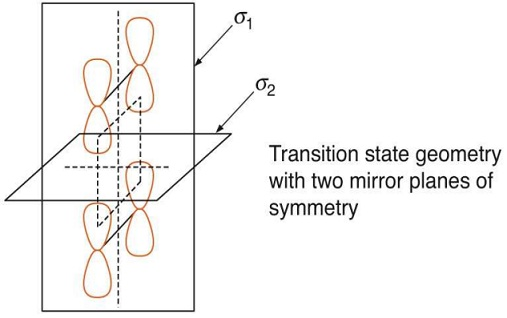Figure: Transition state geometry with two mirror planes of symmetry

The fundamental characteristic of the orbital symmetry analysis is to draw the MOs of the reactant(s) and the MOs of the product(s). One then considers how the symmetries of the orbitals relate to each other. Orbitals of reactants are renovated smoothly into orbitals of products, through conservation of orbital symmetry.

Analysis of cycloaddition reaction by orbital correlation diagram method

Consider the [2+2] cycloaddition of two ethylene molecules. Let us assume that the two molecules approach each other through their π systems arranged symmetrically (for example, a symmetric pericyclic transition state). If so, then we can identify 2 symmetry elements: two mirror planes, as shown. Each pair of interacting pi orbitals in the reactant amalgamation leads to one σ bond in the cyclobutane product.

Figure describes the situations implied via the term head-to-head, namely that the reactants approach each other in parallel planes through the pi orbitals overlapping in the head-to-head fashion needed for the formation of sigma bonds. The sigma-bonded atoms of each ethene lie in the 2 parallel planes exposed in black in the figure. The p orbitals on each carbon lie in the vertical plane that is revealed in blue. The two planes revealed in red are symmetry planes. Plane 1 bisects the C-C bond of each ethene, while plane 2 lies half way between the 2 planes exposed in black.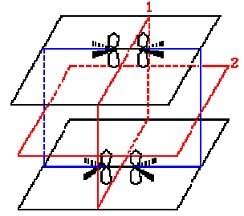According to the conventions of orbital symmetry theory, the reaction exposed in figure is classified as a  π2s +  π2s cycloaddition, where  π specifies that the reaction engages a  π system, the number 2 is the number of electrons in the reacting π system, and the letter s stands for suprafacial: if one lobe of a p orbital is considered as the top face, while the other lobe is said the bottom face, then a suprafacial communication is one in that the bonding happens on the similar face at both ends of the π system. The orientation of the interacting ¼ systems symbolized in Figure implies something about the stereochemical outcome of the reaction. Consider the π2s + π2s cycloaddition revealed in Equation, where the two new sigma bonds are exposed in red.

Since the interacting π systems approach each other in a "head-to-head" fashion, the relative stereochemistry is the similar in the products as it is in the reactants, for instance the 2 carboxyl groups that are 'cis' to each other in the alkene are 'cis' to each other in the products. The alternative to a suprafacial interaction is an antarafacial reaction: in this case bonding happens on the top face at one end of the π system and on the bottom face of the other. We will think such thoughts once more when we talk about the Diels-Alders reaction. Molecular orbital theory explains the formation of the product in reaction 2 in terms of linear combinations the molecular orbitals of the reactants. Each reactant has two pi molecular orbitals of interest, ψ1 and ψ2. There are four amalgamations of these pi orbitals possible: ψ1A + ψ1B, ψ1A - ψ1B, ψ2A + ψ2B, and ψ2A - ψ2B, where the subscripts A and B are used to distinguish one ethene from the other. Such amalgamations convert into the 4 sigma molecular orbitals in the product: σ1A + σ1B, σ1A - σ1B, σ2A + σ2B, and σ2A - σ2B. The diagram depicting the correlation of the reactant and product orbitals is exposed in figure. The numbers 1 and 2 in the diagram refer to the symmetry planes 1 and 2 in Figure.

Analysis of electrolytic reaction by orbital correlation diagram method

Example 1: Interconversion of cyclobutene to butadiene. For the disrotatory procedure not all the orbitals correlate through ground state orbitals of butadiene. The subsequent conclusions can be drawn from the correlation diagram.

1. We imagine a thermal transformation to happen only when the ground state orbitals of cyclobutene correlate through the ground state orbitals of butadiene. Even though σ correlates with π1, the π orbital of cyclobutene isn't correlating with π2 of butadiene. Therefore thermal alteration of cyclobutene to butadiene via disrotatory procedure is equilibrium forbidden.

2. Irradiation of cyclobutene causes excitation through electron distribution σ2, π1, π*1 that correlates by 1st excited state orbitals of butadiene. The photochemical procedure is symmetry permitted.

Example 2: Conrotaory ring opening of cyclobutene -butadiene -C2 symmetry. From the correlation diagram 2 conclusions can be drawn:

1. since there are correlation between the ground state orbitals of cyclobutene and butadiene.  A thermal conrotatory procedure is symmetry permitted.

2. The first excited state of cyclobutene (σ2, π1, π*1) correlates by not first excited state but an upper excited state of butadiene the process is symmetry forbidden. Similarly the first excited state of butadiene is correlating through a higher energy upper excited state of cyclobutene for instance, a photochemical conrotatory procedure in either direction is symmetry forbidden.

On similar grounds we can work out the disrotatory and conrotatory modes interconversion of hexatriene - cyclohexadiene. It can be noted that the thermal disrotatory and photochemical conrotatory processes are symmetry allowed. Such generalizations are Woodward -Hoffmann rules for electro cyclic reactions that can be summarized as

Frontier Molecular Orbital Theory

Frontier Molecular Orbital Theory was expanded in the year 1960s via Kenichi Fukui who recognized, which chemical reactivity can frequently be described in terms of interacting Highest Occupied MOs (HOMOs), Lowest Unoccupied MOs (LUMOs) and Singly Occupied MOs (SOMOs).

• HOMO + LUMO -> bonding MO
• HOMO + HOMO -> antibonding MO
• LUMO + LUMO -> null interaction (no electrons)
• SOMO + SOMO -> bonding MO

The FMO approach was developed by Woodward & Hoffmann in the late nineteen sixties who utilized it to explicate an apparently diverse set of reactions involving π-systems, involving Diels-Alder cycloaddition. Hoffmann utilized the approach to discover transition metal compounds. According to Frontier Orbital Theory it is possible to agree on if a pericyclic reaction is permitted or forbidden via merely considering the symmetry association of the frontier orbitals of the reactants. The frontier orbitals are the highest occupied molecular orbital (HOMO) and the lowest unoccupied molecular orbital (LUMO). The communication between such orbitals, a so-said HOMO-LUMO interaction, is an idea that is similar to Lewis acid-Lewis base chemistry that involves the interaction of a filled orbital of the base through an empty orbital of the acid. According to Frontier Orbital Theory, a pericyc lic reaction is allowed when the HOMO of one reactant has the similar symmetry as the LUMO of the other. The bonding or antibonding contacts of the frontier molecular orbital(s) determine whether the reactions are thermally or photochemically allowed or forbidden. Cycloaddition can be described using frontier molecular orbital (FMO) theory. The alkene (dienophile) component has 2 electrons is a "single" π-bond. FMO theory, here, identifies the HOMO and LUMO components of this system: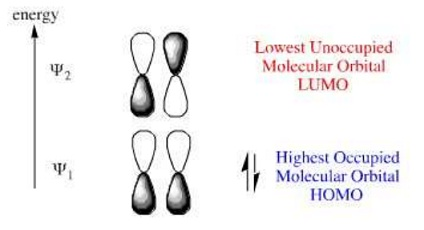Figure: HOMO/LUMO components of a dienophile

Likewise, the diene which has four electrons in its conjugated π-system can have its HOMO and LUMO identified within FMO theory: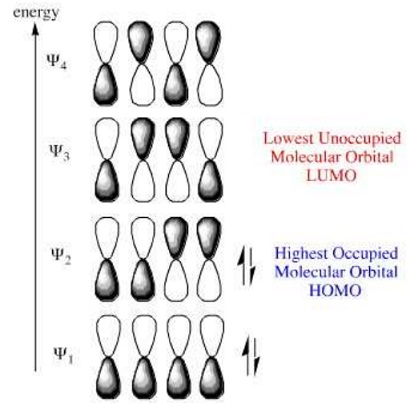Figure: HOMO/LUMO components of a diene

If we examine the phases at the ends (termini) of the diene and dieneophile we discover that the LUMO/HOMO interactions are phase matched: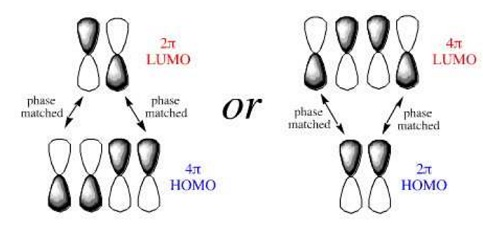Figure: LUMO/HOMO interactions of diene and dienophile

Notice that the phases match whichever species is defined as the HOMO or LUMO. In reality, the electron rich species reacts via its HOMO and the electron poor species via its LUMO. In the FMO diagrams above, the sizes or coefficients are all the similar size, but generally they are of different sizes. The rule is that the coefficients match as well: small through small and huge by huge: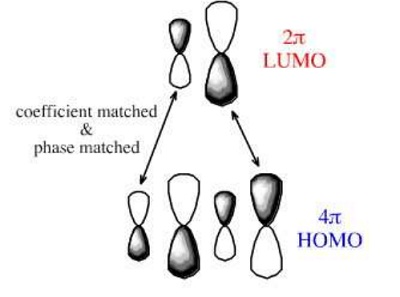Figure: Coefficient and phase matched HOMO/LUMO

We can utilize Hückel MO theory to compute the sizes of the coefficients at each of the atoms. (There is a web based HMO calculator, here, even though at meta-synthesis we employ a standalone package: HMO by Allan Wissner.) If the coefficients are computed for 1-methoxy-1,3-butadiene the termini are +0.3 and -0.58 (or -0.3 and and +0.58). For acrylonitrile the coefficients are +0.2 and -0.66 (or -0.2 and +0.66).

The cycloaddition reaction proceeds so the coefficients 'match', both in terms of phase (essential) and in terms of coefficient magnitude: +0.3 with +0.2 and -0.58 with -0.66. Therefore, the regioselectivity of the cycloaddition can be explained: Fukui realized that a good approximation for reactivity could be originated via looking at the frontier orbitals (HOMO/LUMO). This was depends on 3 major observations of molecular orbital theory as 2 molecules interact:

1.  The occupied orbitals of different molecules repel each other.

2.  Positive charges of one molecule attract the negative charges of the other.

3.  The occupied orbitals of one molecule and the unoccupied orbitals of the other (especially the HOMO and LUMO) interact through each other causing attraction. From such observations, frontier molecular orbital (FMO) theory simplifies reactivity to interactions between the HOMO of one species and the LUMO of the other. This assists to describe the calculations of the Woodward-Hoffmann rules for thermal pericyclic reactions that are summarized in the subsequent statement: "A ground-state pericyclic change is symmetry-allowed when the total number of (4q+2)s and (4r)a components is odd" (4q+2)s refers to the number of aromatic, suprafacial electron systems; likewise, (4r)a refers to antiaromatic, antarafacial systems. It can be revealed that if the total number of such systems is odd then the reaction is thermally permitted.

Analysis of Electrocyclic reactions by FMO

Electrocyclic reactions, like all pericyclic processes, exhibit huge stereoselectivity. Consider two 1,3,5-hexatriene systems embedded into longer hydrocarbon chains.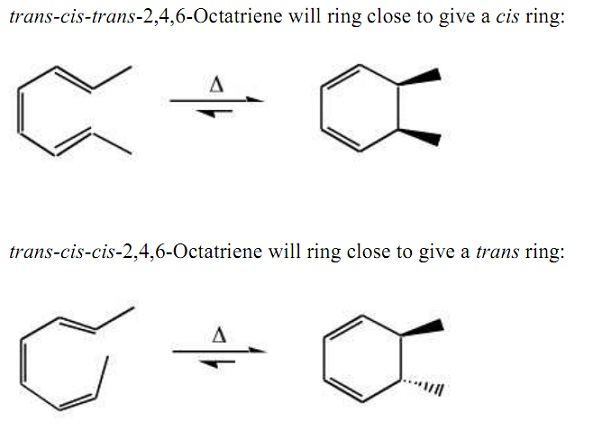As with cycloaddition, this selectivity can be explained by examining the FMOs, specifically the HOMO: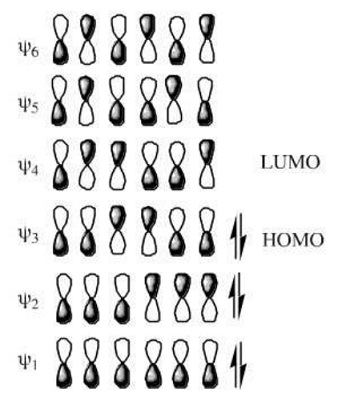Figure: Superimposition of HOMO upon triene system

If the termini of the HOMO are superimposed upon the triene system, it can be seen that the end groups must rotate in a disrotatory manner (twist in opposite directions, when viewed front-on) to shape the bond: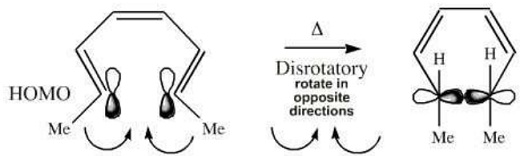Figure: Disrotatory twist of HOMO

However, electrocyclic reactions can also occur photochemically. When photoactivated, an electron moves from the HOMO to the next orbital, the LUMO. (Now this orbital contains an electron it is no longer unoccupied, it is either a SOMO or an excited state HOMO). The photoexcited system will ring-close in the opposite manner to the thermal system and the groups conrotate (twist the same way) to form the sigma bond: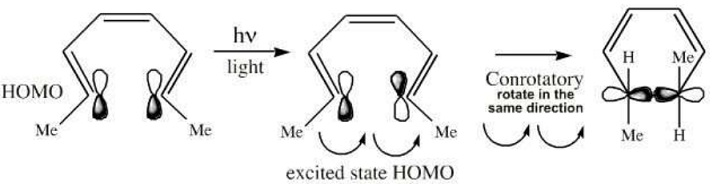Figure: Conrotation of photoexcited System

The 2 products have dissimilar stereochemistry and are diastereomers. This thermal and photo selectivity can be exploited in the reaction sequence below in that 1,3,5-cyclononatriene is changed into bicyclic systems, first with  cis and then trans ring junctions. The initial 1,3,5-cyclononatriene is all  cis. This is thermally ring closed in a disrotatory manner and then photo-ring opened at -20°C in a conrotatory manner. The 1,3,5-cyclononatriene now has two cis and one trans double bonds however, the nine membered ring is able to accommodate the strain of a trans alkene. Thermal ring closure provides the trans-ring junction.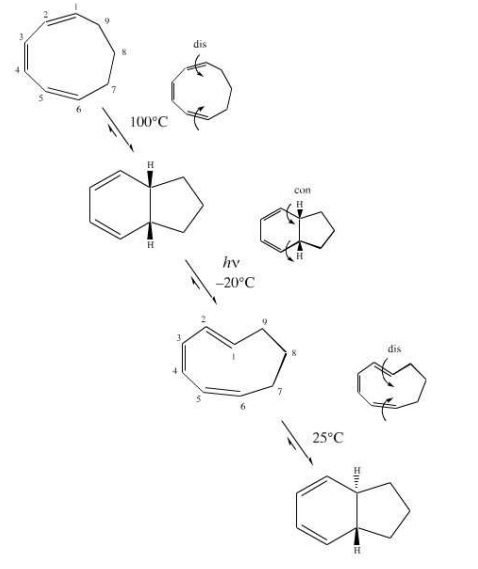Figure: Conrotatory twist of 1,3,5-cyclononatriene

Reaction conditions for pericyclic reactions

It is examined, which several of the pericyclic reactions take place only upon heating, whereas others are probable only under photochemical conditions for example the Diels-Alder reaction, a [4+2] cycloaddition happens under thermochemical situations and isn't possible under photochemical conditions.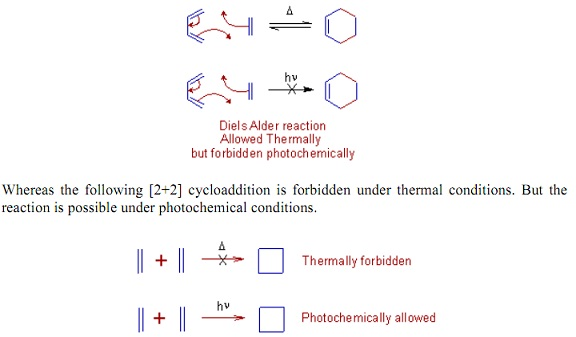Fig: Diels alder reaction

Woodward-Hoffmann rules

To expect whether a pericyclic reaction is permitted or not under specified situation, Woodward and Hoffmann proposed subsequent set of regulations Woodward-Hoffmann rules idea. A thermal pericyclic reaction is permitted in the ground state, when the total number of (4q + 2)s and (4r)a components is odd. Or else, if the total of (4q + 2)s and (4r)a components is even, the pericyclic reaction is allowed in the stimulated state for example, under photochemical situations.

Table Woodward-Hoffmann rules for predicting allowed pericyclic reaction

 Number of (4q + 2)s and (4r)a components The condition under which the reaction is allowed Odd Thermal even photochemical

Component: A bond(s) or an orbital(s) taking part in the pericyclic reaction as a single unit can be considered as a component. It can have any number of electrons but might not contain mixtures of π and σ electrons. For illustration a double bond is considered as a π2 component, because there are two π electrons. A conjugated diene can be considered as π4 component, as there are four π electrons. 's' symbolizes suprafacial. A suprafacial component forms new bonds on the similar face at its both ends. 'a' symbolizes antarafacial. An antarafacial component shapes new bonds on the opposite faces of its together finishes. For example π2s symbolizes a component enclosing 'two π electrons and forming new bonds in suprafacial manner. π4a symbolizes a component enclosing four π electrons and is going to form new bonds in antarafacial manner.

q & r: Such are integers.

(4q + 2)s component: The suprafacial component that might have either 2 or 6 or 10 or _ _ _ electrons of similar kind. Such numbers are attained via substituting 'q' by 0 or 1 or 2 or _ _ _.

(4r)a component: The antarafacial component that might have either 4 or 8 or 12 or _ _ _ electrons of similar kind. Such numbers are attained via substituting 'r' by 1 or 2 or 3 or _ _ _.

Similarly the meanings of (4q + 2)a & (4r)s can be understood.

Application: Let us assume the diene and dienophile in Diels-Alder reaction are approaching suprafacially as revealed below.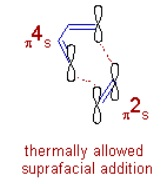Since there are 4 π electrons in diene that is making bonds in suprafacial manner it is a (4q + 2)s component. For instance, there is one (4q + 2)s component. And the alkene is a (4r)s component, because it has 2 π electrons and is approaching the diene suparfacially for example, there are no (4r)a components. Therefore, the total number of (4q + 2)s and (4r)a components = 1 + 0 = 1, an odd number. Therefore Diels-Alder reaction is thermally permitted in ground state when both the components are approaching suprafacially. Therefore it is said as π4s + π2s cycloaddition. Antarafacial addition isn't allowed under thermal conditions but is theoretically allowed under photochemical situations in the excited state. Though, the strain in the transition state while do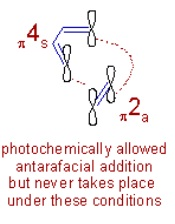ing so forbids to do so.

As we know the orbitals revealed in above diagrams are simple 'p' orbitals and aren't the frontier molecular orbitals. Don't mix explanations of FMO theory through Woodward-Hoffmann rules.

Simplified Woodward-Hoffmann rules

Application of above Woodward-Hoffmann rules to pericyclic reactions is tedious and cumbersome; subsequent simplified rules can be utilized to predict theoretically permuted modes of pericyclic reactions under specified situations.

Table Simplified Woodward-Hoffmann rules

 No. of π electrons Reaction conditions Allowed mode (4n+2) A Huckel number Thermal Supra (or) Dis Photochemical Antara (or) Con (4n) A non Huckel number Thermal Antara (or) Con Photochemical Supra (or) Dis

Remember that even though the pericyclic reactions are permitted theoretically under both the conditions in either of the modes, sometimes the factors as steric obstruction and strain in the evolution state might forbid the reaction to take place.

Table Pericyclic Reaction Rules

 Designation Thermal Photochemical π2s + π2s forbidden allowed π2s + π4s a allowed forbidden π2s + π2a allowed forbidden π2s + π4a f forbidden allowed π2a + π2a forbidden allowed π2a + π4a allowed forbidden

Before we move on, it is worthwhile to clarify the implications of the expressions permitted and forbidden. An allowed reaction is merely one through a low activation relative to several other pathways while a forbidden reaction is a procedure for that there is important activation energy. In expressions of transition states, an allowed reaction proceeds via an aromatic transition state, while a forbidden reaction doesn't take place since the transition state would be "anti-aromatic."

Tutorsglobe: A way to secure high grade in your curriculum (Online Tutoring)